# #389 Two-stage Common Emitter Amplifier

Designing a two-stage common-emitter BJT amplifier.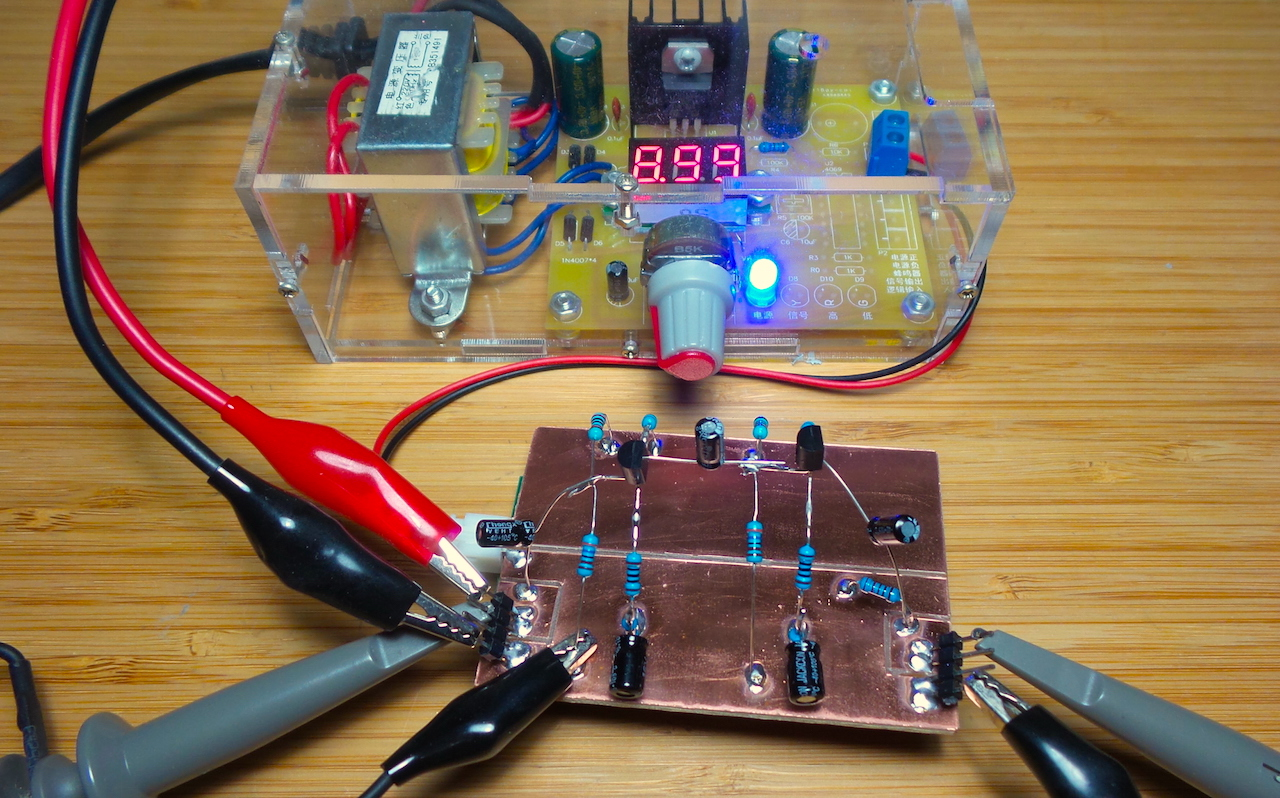Cascading two common-emitter amplifiers is a means of achieving high voltage gain. Voltage gains from several hundred to several thousand are possible.

I’m going to try and noodle out a theoretical design of a two-stage Class A amplifier, and then test the actual performance. Do not take my calculations as gospel. My main sources for the theory were Electronic Principles by Albert Paul Malvino and a Multistage Transistor Amplifiers YouTube tutorial by The Offset Volt:### Designing a Single Stage for Gain ~ 10

Parameter Design/Spec Value
A (gain) 10
Vcc 9V
Icq 4mA
Vceq 4.5V
ß (hFE) 100 - 400
hie 1kΩ - 10kΩ
Vbe 0.7V
RL 2.2kΩ

Also:

• r’e = hie/hFE = 10kΩ/400 = 25Ω

#### Calculate collector + emmiter resistance for desired gain at the Q point

• Rc + Re = (Vcc - Vceq) / Icq
• Rc + Re = (9V - 4.5V)/4mA = 1.13kΩ
• assuming A ≅ Rc/Re
• Re = 1.13kΩ - Rc
• Re = 1.13kΩ/11 =100Ω
• Rc = 1.03kΩ, say 1kΩ

With the selected components, the theoretical gain is thus 10

#### Calculate the combined bias gang resistance

Base current at the q point

• Ib = 4mA / 100 = 0.04mA

Assume current through the gang at 10 x Ib as a rule of thumb to ensure “stiff” biasing i.e. 0.4 mA

So combined resistance = 22.5kΩ

#### Calculate the resistance of R1 and R2 components of the bias gang

Lower resistor R2:

voltage = 0.7 + Ic x Re = 1.1V

therefore R2 = 2.75kΩ say 3kΩ (standard value)

and therefore R1 = 19.5kΩ say 20kΩ (standard value)

#### Measure Performance - Single Stage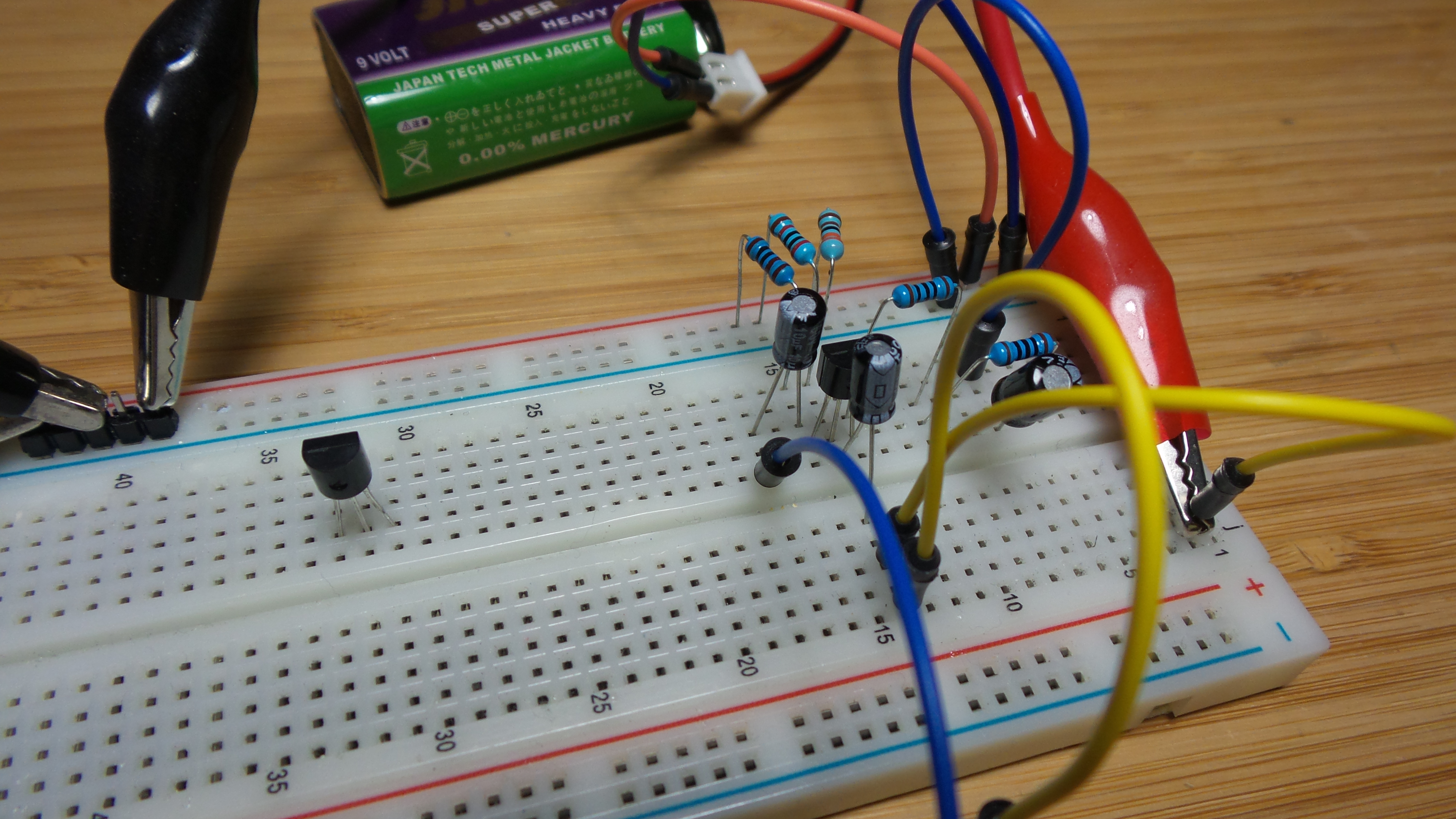With 0.2V peak-peak 10kHz input, and Re = 100Ω (without emitter bypass), measured results:

• 0.2V input peak-peak
• 1.98V output peak-peak
• actual gain = 9.9

Conclusion: very close to design gain of 10, undistorted Class A performance.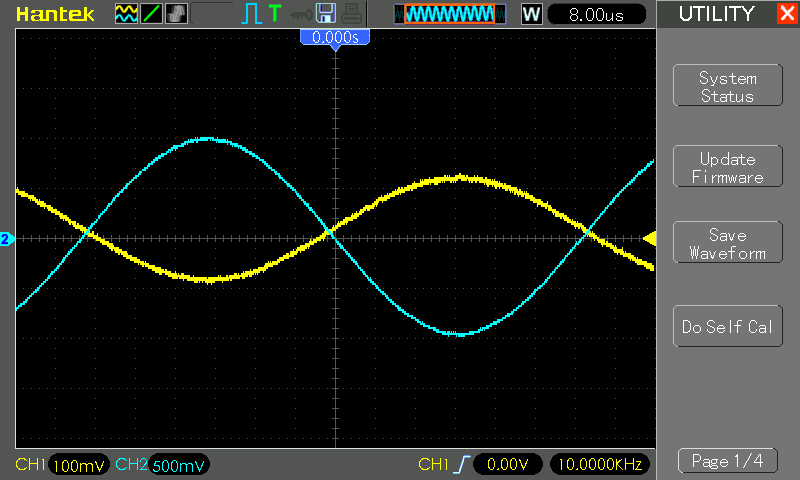### Adjusting Single Stage Design with Bypass

I decided to add another 100Ω/100µF emitter bypass to the design, for a few reasons:

• it’s a common feature of such designs
• it provides negative feedback to stabilise for transistor variations (AC signals will vary the emitter resistance with inverse relationship to input/output differential, thus combating variation)
• reduces the gain a bit (so I can work with larger input signals in two-stage configuration while staying within the 9V supply limits)

Recalculating with this variation. DC characteristics are now:

• Re = 200Ω
• A ≅ Rc/Re = 1kΩ/200Ω = 5

combined base bias resistance should still be = 22.5kΩ

Lower resistor R2 is now:

voltage = 0.7 + Ic x Re = 1.5V

therefore R2 = 3.75kΩ say 3.6kΩ (standard value)

and therefore R1 = 18.9kΩ say 20kΩ (standard value)

### Calculating Two-stage Performance with RL load

With:

• component values duplicated the from the single-stage design for two stages
• with the extra `100Ω||100µF` emitter bypass
• and RL = 2.2kΩ

predicted performance calculated as follows:

DC analysis:

• Vb = Vcc*Rb2/(Rb1 + Rb2) = 9V * 3.6kΩ/(20kΩ + 3.6kΩ) = 1.37V
• Ve = Vb - 0.7V = 0.67V
• Ie = Ve/Re = 0.67V/(200Ω) = 3.35mA
• Ie ≅ Ic
• Vrc = Ic * Rc = 3.35mA * 1kΩ = 3.35V
• Vce = Vcc - (Vrc + Ve) = 9V - (3.35V + 0.67V) = 4.98V
• Isat = Vcc/(Re + Rc) = 9V/(200Ω + 1kΩ) = 7.5mA

Second stage:

• assuming thermal voltage VT = kT/q = 25 mV
• r’ej = VT/Ie = 7.5Ω
• A2 = `Rc||RL/(Re2 + r'ej)` = `1k||2.2kΩ/(100Ω + 7.5Ω)` = 6.4
• Rin(base) = ß(Re2 + r’ej) = 100(100Ω + 7.5Ω) = 10.75kΩ
• Zin = `Rin(base)||Rb1||Rb2` = `10.75kΩ||20kΩ||3.6kΩ` = 2.38kΩ
• A1 = `Rc1||Zin/(Re1 + r'ej)` = `1kΩ||2.38kΩ/(100Ω + 7.5Ω)` = 6.55
• Total gain A = A1 * A2 = 6.4 * 6.55 = 41.92

Assuming headroom for say 7V peak-peak, input limit would be around 0.17V peak-peak before clipping.

#### Measure Performance - Two Stage

With 0.1V peak-peak 10kHz input, and Re = 200Ω (with 100Ω/100µF bypass), measured results:

• 0.1V input peak-peak
• 3.88V output peak-peak
• actual gain = 38.8 = 31.8 dB
• error in the theoretical gain calc = 7%

Conclusion: predicted performance was quite close to the actual performance. And .. I have a functioning two-stage Class A amplifier to boot, with performance “in the ballpark” of the design.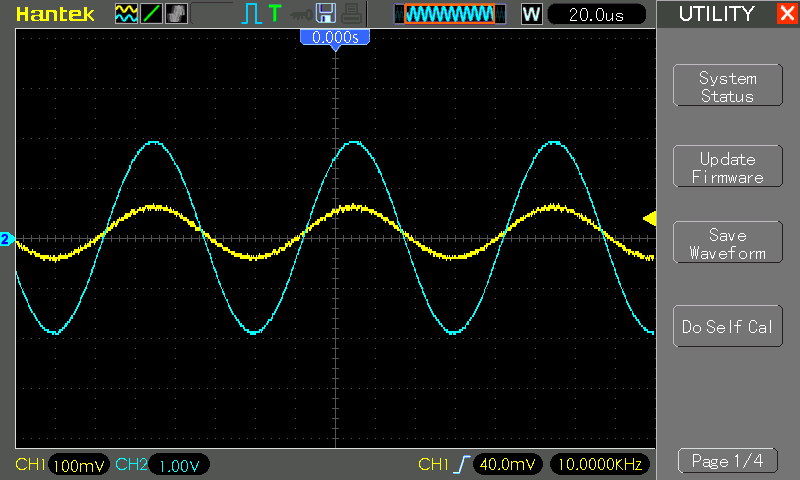### Performance With an Ugly-style Build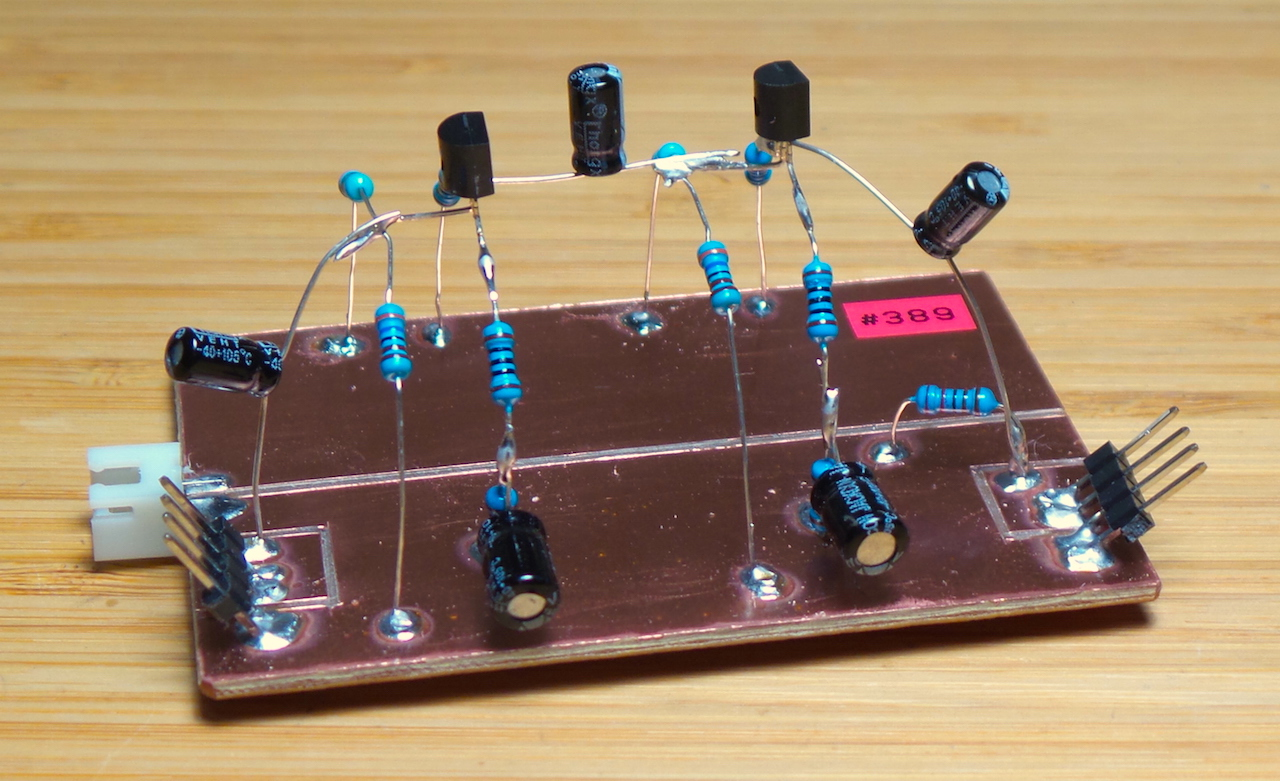With an ugly-style build, I see even better performance, and improved gain:

• 0.1V input peak-peak
• 4.32V output peak-peak
• actual gain = 43.2 = 32.71 dB
• error in the theoretical gain calc = -3%

More gain when built “properly”, this time overshooting estimates by a small margin (less than the tolerance on components).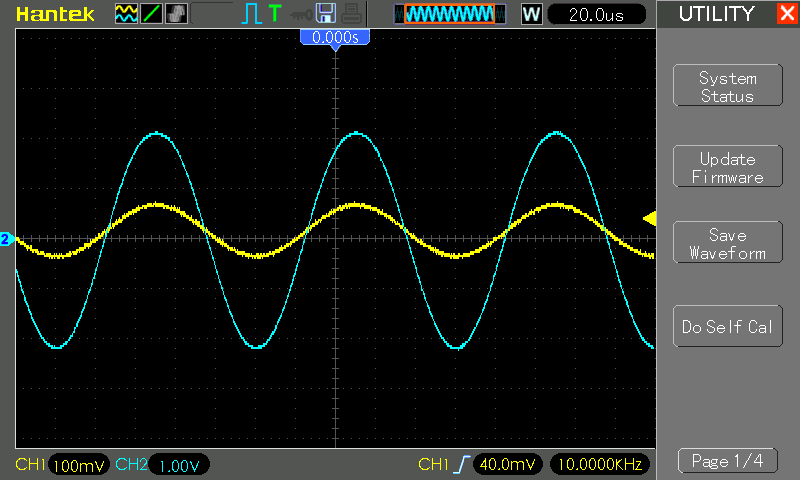At 400kHz, perfromance is maintained: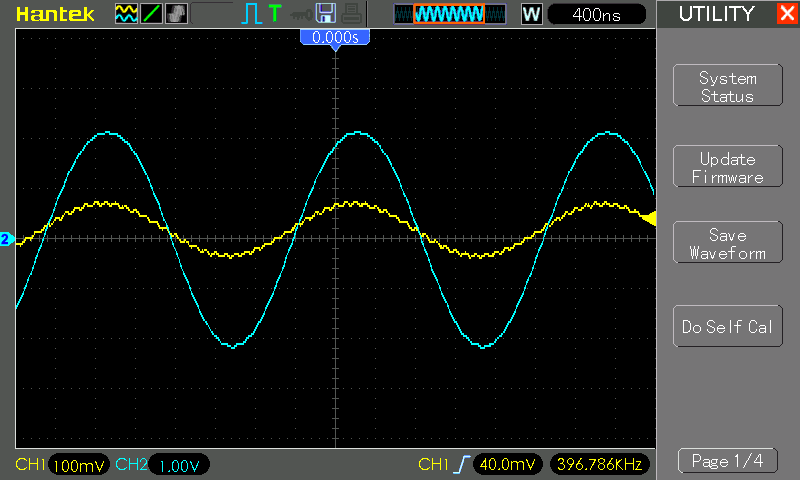## Construction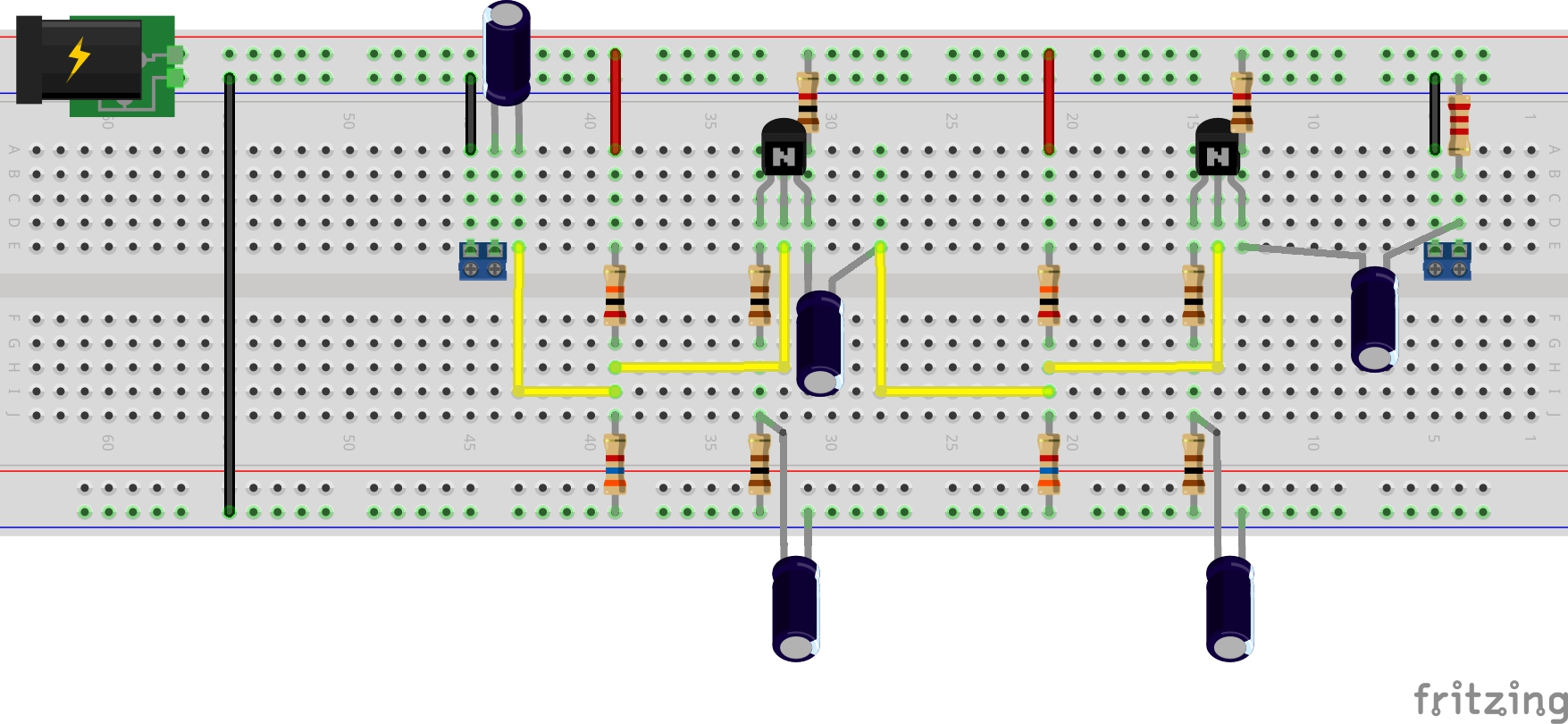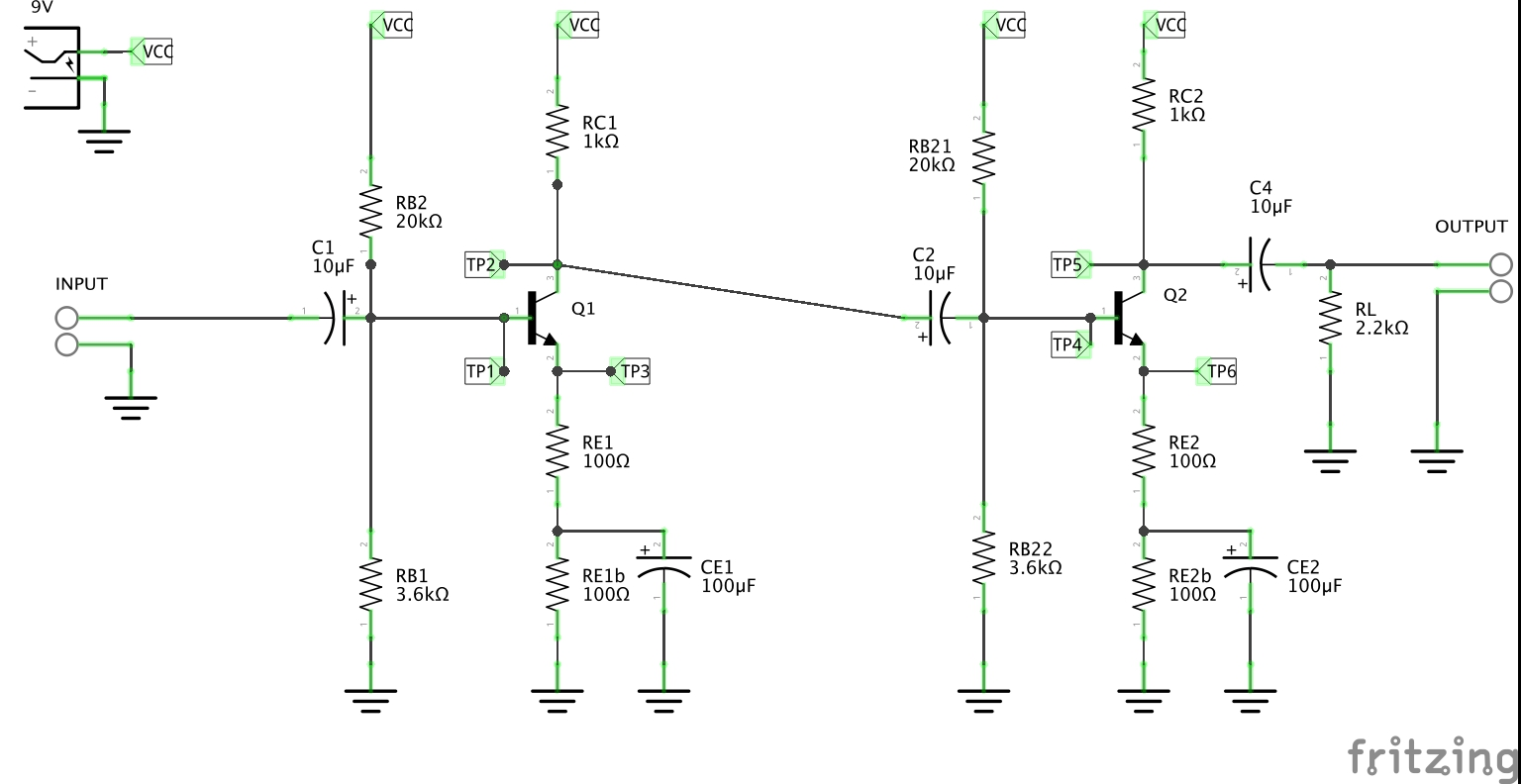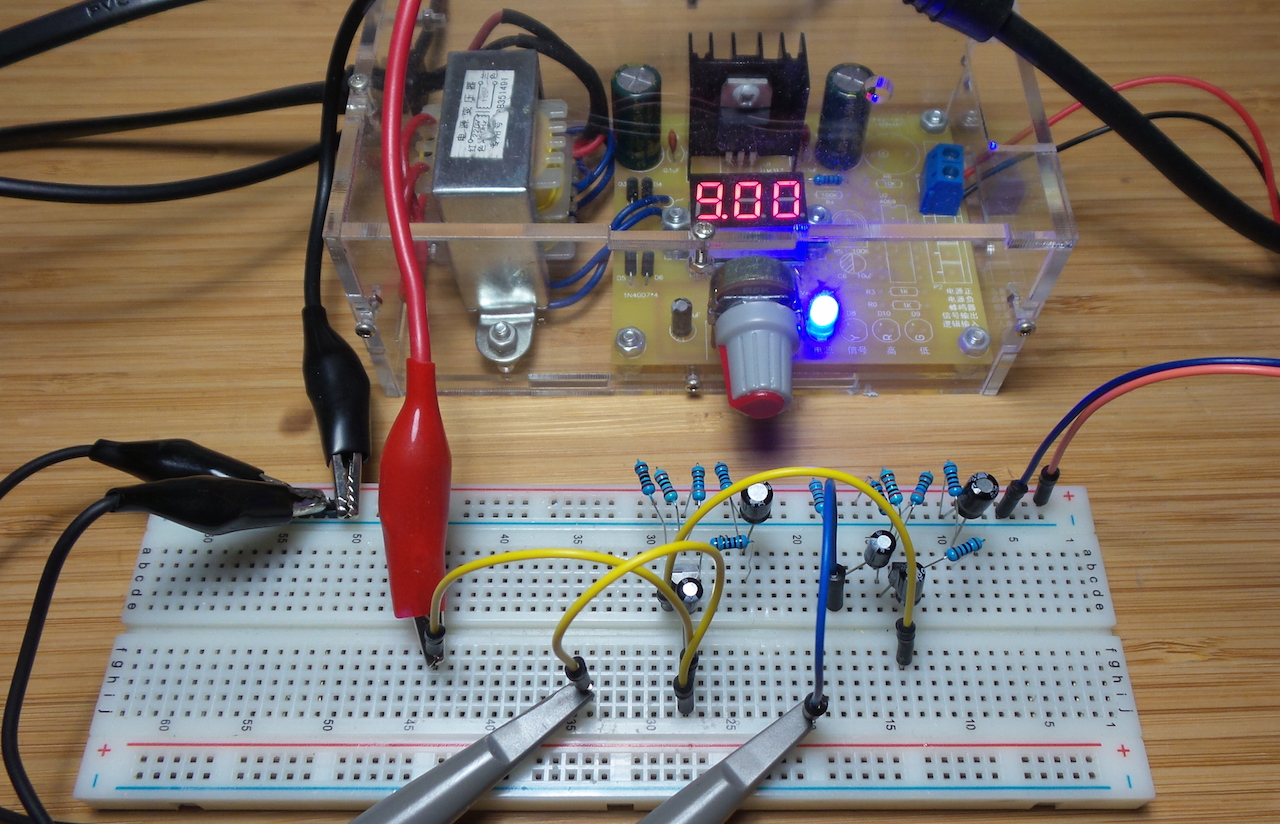Testing with an ugly-style build: